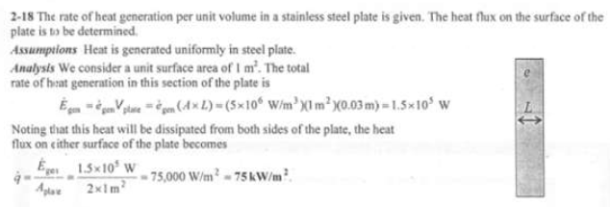heat and mass transfer problems engineering equations heat and mass transfer school homework engineering heat and mass transfer formulas heat and mass transfer solutions to heat and mass transfer problems full solution engineering problem solution heat and mass transfer math problems engineering equations heat and mass transfer school homework engineering solutions to heat and mass transfer formulas heat problems mass problem solutions to transfer problems full solution heat and mass transfer
heat and mass transfer problems engineering equations heat and mass transfer school homework engineering heat and mass transfer formulas heat and mass transfer solutions to heat and mass transfer problems full solution engineering problem solution heat and mass transfer math problems engineering equations heat and mass transfer school homework engineering solutions to heat and mass transfer formulas heat problems mass problem solutions to transfer problems full solution heat and mass transfer
Highalphabet Home Page heat and mass transfer problem solutions Heat and Mass Transfer Page
Consider a large 3-cm-thick stainless steel plate in which heat is generated uniformly at a rate of 5x10^6 W/m^3. Assuming the plate is losing heat from both sides, determine the heat flux on the surface of the plate during steady operation.Consider a large 3-cm-thick stainless steel plate in which heat is generated uniformly at a rate of 5x10^6 W/m^3. Assuming the plate is losing heat from both sides, determine the heat flux on the surface of the plate during steady operation.